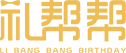• A
• B
• C
• D
• E
• F
• G
• H
• J
• K
• L
• M
• N
• P
• Q
• R
• S
• T
• W
• X
• Y
• Z

# 女朋友生日可以送什么礼物？\$(function(){ if( \$('.flower-l-content-text').html().indexOf('[AD]')>0 ){ \$('.flower-l-content-text').html(function(i, h){ if( h.indexOf('[AD]') ) h = h.replace( '[AD]', \$('#sp1').html() ); if( h.indexOf('[AD]') ) h = h.replace( '[AD]', \$('#sp2').html() ); if( h.indexOf('[AD]') ) h = h.replace( '[AD]', \$('#sp3').html() ); return h; }); } else{ var p = \$('.flower-l-content-text p').length; \$('.flower-l-content-text p').eq( Math.ceil(p*0.2) ).after( \$('#sp1').html() ); \$('.flower-l-content-text p').eq( Math.ceil(p*0.5) ).after( \$('#sp2').html() ); \$('.flower-l-content-text p').eq( Math.ceil(p*0.8) ).after( \$('#sp3').html() ); } });Roselove微笑星眸花束Roselove灿若云霞花束“Lovely angel可爱天使”-\$(function(){ if( \$('.flower-l-content-text').html().indexOf('[AD]')>0 ){ \$('.flower-l-content-text').html(function(i, h){ if( h.indexOf('[AD]') ) h = h.replace( '[AD]', \$('#sp1').html() ); if( h.indexOf('[AD]') ) h = h.replace( '[AD]', \$('#sp2').html() ); if( h.indexOf('[AD]') ) h = h.replace( '[AD]', \$('#sp3').html() ); return h; }); } else{ var p = \$('.flower-l-content-text p').length; \$('.flower-l-content-text p').eq( Math.ceil(p*0.2) ).after( \$('#sp1').html() ); \$('.flower-l-content-text p').eq( Math.ceil(p*0.5) ).after( \$('#sp2').html() ); \$('.flower-l-content-text p').eq( Math.ceil(p*0.8) ).after( \$('#sp3').html() ); } });Roselove千娇百媚花束Roselove娇艳魅人枪炮礼盒Roselove娉婷袅袅花束